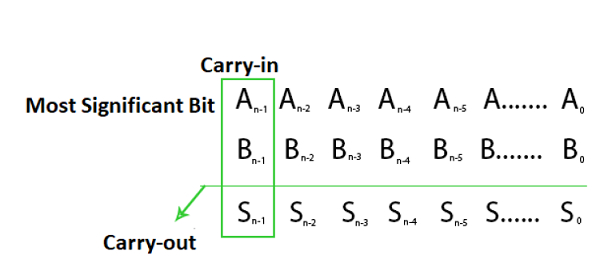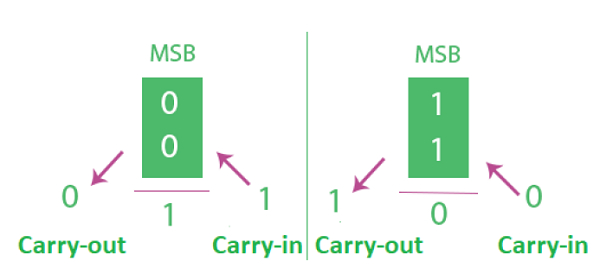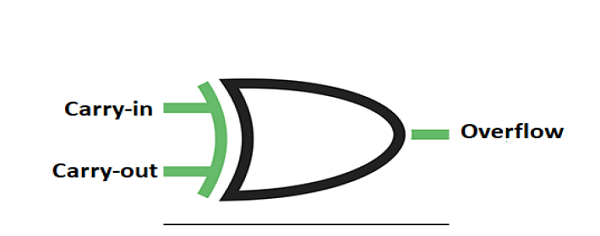# Binary Number System - Overflow in Arithmetic Addition in C/C++?

2’s Complement Number System is widely implemented in computer architecture.

N-bit 2’s Complement number System can be able to represent Number from -2n-1 to 2n-1- 1

4 Bit can be able to represent numbers from ( -8 to 7 )

5 Bit can be able to represent numbers from ( -16 to 15 ) in 2’s Complementary System.

Overflow happens with respect to addition when 2 N-bit 2’s Complement Numbers are appended and the answer is too large to fit into that N-bit Group.

A computer contains N-Bit Fixed registers. Result of addition of two N-Bit Number will result maximum N+1 Bit number.

Carry Flag stores that Extra Bit. But Carry does not always specify overflow.

## Overflow Detection

Overflow happens when −

• Result of addition of two negative numbers is positive or

• Result of addition of two negative numbers is negative.

So overflow can be detected by verifying Most Significant Bit(MSB) of two operands and result. But Instead of implementing 3-bit Comparator Overflow can also be detected implementing 2 Bit Comparator just by verifying Carry-in and Carry-Out from MSB’s. We consider N-Bit addition of 2’s Complement number.Overflow happens when Carry-in not equal to Carry-out. Above expression for overflow can be discussed from below analysis.In case of first Figure the MSB of two numbers are 0 which indicates they are positive. Here if Carry-in is 1 we get result’s MSB as 1 indicates result is negative (Overflow) and Carry-out as 0. Carry-in is not equal to Carry-out hence overflow.

In case of second Figure the MSB of two numbers are 1 which indicates they are negative. Here if Carry-in is 0 we get result MSB as 0 indicates result is positive(Overflow) and Carry-out as 1. Carry-in is not equal to Carry-out hence overflow.

So Carry-in and Carry-out at MSB’s are sufficient to detect Overflow.Above XOR Gate can be implemented to detect overflow.

Updated on: 29-Jan-2020

376 Views